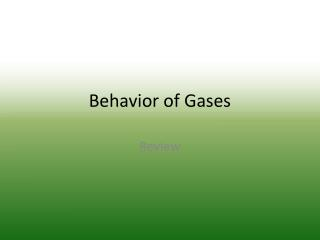DownloadDownload PresentationBehavior of Gases

# Behavior of Gases

Download Presentation## Behavior of Gases

- - - - - - - - - - - - - - - - - - - - - - - - - - - E N D - - - - - - - - - - - - - - - - - - - - - - - - - - -
##### Presentation Transcript

1. Behavior of Gases Review

2. True or False: One mole of any gas, regardless of size, temperature, or pressure occupies 22.4L? • True • False

3. True or False: STP is at 0 Kelvin and 760mmHg. • True • False

4. Which of the following is not a main idea of the kinetic molecular theory of gases? • The average kinetic energy of gas particles is proportional to the Kelvin temperature. • Gases consist of molecules moving at extremely high velocities. • STP is at 0o C and 1atm. • The volume of the individual gas molecules is minuscule compared to the volume that the gas occupies.

5. If the total atmospheric pressure is 760mmHg, then all of the components of the air (nitrogen, oxygen, …) have a pressure of 760mmHg. • True • False

6. Why is a gas easier to compress than a liquid or a solid? • Its volume increases more under pressure than an equal volume of liquid does. • Its volume increases more under pressure than an equal volume of solid does. • The space between gas particles is much less than the space between liquid or solid particles. • The volume of a gas’s particles is small compared to the overall volume of the gas.

7. How does the gas propellant move when an aerosol can is used? • from a region of high pressure to a region of lower pressure • from a region of high pressure to a region of equally high pressure • from a region of low pressure to a region of higher pressure • from a region of low pressure to a region of equally low pressure

8. If a balloon is squeezed, what happens to the pressure of the gas inside the balloon? • It increases. • It stays the same. • It decreases. • The pressure depends on the type of gas in the balloon.

9. As the temperature of the gas in a balloon decreases, which of the following occurs? • The volume of the balloon increases. • The average kinetic energy of the gas decreases. • The gas pressure inside the balloon increases. • all of the above

10. If 4 moles of gas are added to a container that already holds 1 mole of gas, how will the pressure change inside the container? • The pressure will be five times higher. • The pressure will double. • The pressure will be four times higher. • The pressure will not change.

11. Which of these changes would NOT cause an increase in the pressure of a contained gas? • The volume of the container is increased. • More of the gas is added to the container. • The temperature is increased. • The average kinetic energy of the gas in increased.

12. When the Kelvin temperature of an enclosed gas doubles, the particles of the gas ____. • move faster • strike the walls of the container with less force • decrease in average kinetic energy • decrease in volume

13. The volume of a gas is doubled while the temperature is held constant. How does the gas pressure change? • It is reduced by one half. • It does not change. • It is doubled. • It varies depending on the type of gas.

14. In general, for a gas at a constant volume, ____. • the pressure of the gas is inversely proportional to its temperature in kelvins • the volume of the gas is inversely proportional to its temperature in kelvins • the volume of the gas is directly proportional to its temperature in kelvins • the pressure of the gas is directly proportional to its temperature in kelvins

15. What happens to the partial pressure of oxygen in a sample of air if the temperature is increased? • It increases. • It stays the same. • It decreases. • The change cannot be determined.

16. If oxygen is removed from a sample of air as iron rusts, what happens to the total pressure of the air? • It increases. • It stays the same. • It decreases. • The change cannot be determined.

17. If the volume of a container of air is reduced by one-half, what happens to the partial pressure of oxygen within the container? • It is reduced by one-half. • It does not change. • It is doubled. • It is reduced by one-fourth.